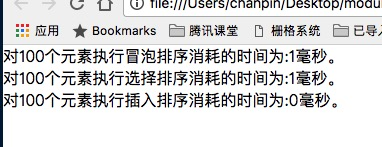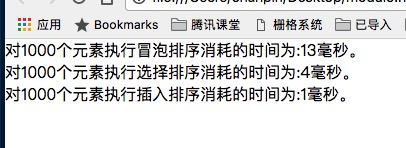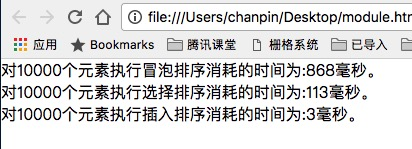# 基本排序算法

``````function CArray(numElements) {
this.dataStore = [];
this.pos = 0;
this.numElements = numElements;
this.insert = insert;
this.toString = toString;
this.clear = clear;
this.setData = setData;
this.bubbleSort = bubbleSort;
this.swap = swap;
for ( var i = 0; i < numElements; ++i ) {
this.dataStore[i] = i;
}
}

function setData() {
for ( var i = 0; i < this.numElements; ++i ) {
this.dataStore[i] = Math.floor(Math.random() * (this.numElements + 1));
}
}

function clear() {
for ( var i = 0; i < this.dataStore.length; ++i ) {
this.dataStore[i] = 0;
}
}

function insert(element) {
this.dataStore[this.pos++] = element;
}

function toString() {
var retstr = "";
for ( var i = 0; i < this.dataStore.length; ++i ) {
retstr += this.dataStore[i] + " ";
if (i > 0 & i % 10 == 0) {
retstr += "\n";
}
}
return retstr;
}

function swap(arr, index1, index2) {
var temp = arr[index1];
arr[index1] = arr[index2];
arr[index2] = temp;
}
``````

• 插入新数据
• 显示数组数据
• 调用不同的排序算法
• 交换数组元素

#### 冒泡排序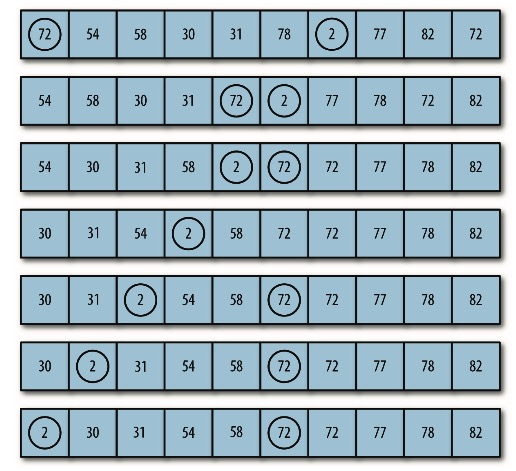``````function bubbleSort() {
var numElements = this.dataStore.length;
var temp;
for ( var outer = numElements; outer >= 2; --outer) {
for ( var inner = 0; inner <= outer - 1; ++inner ) {
if (this.dataStore[inner] > this.dataStore[inner + 1]) {
swap(this.dataStore, inner, inner + 1);
}
}
}
}
``````

``````var numElements = 10;
var mynums = new CArray(numElements);
mynums.setData();
document.write(mynums.toString());
mynums.bubbleSort();
document.write('<br />');
document.write(mynums.toString());
``````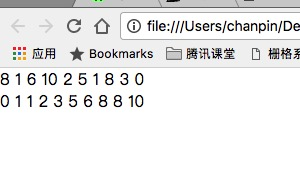``````function bubbleSort() {
var numElements = this.dataStore.length;
var temp;
for (var outer = numElements; outer >= 2; --outer) {
for (var inner = 0; inner <= outer - 1; ++inner) {

if (this.dataStore[inner] > this.dataStore[inner + 1]) {
swap(this.dataStore, inner, inner + 1);
}
}
document.write('<br />');
document.write(this.toString());
}
}
``````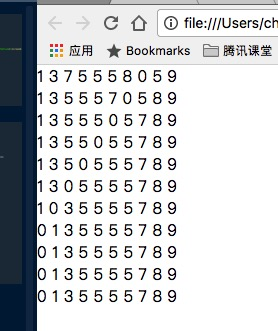#### 选择排序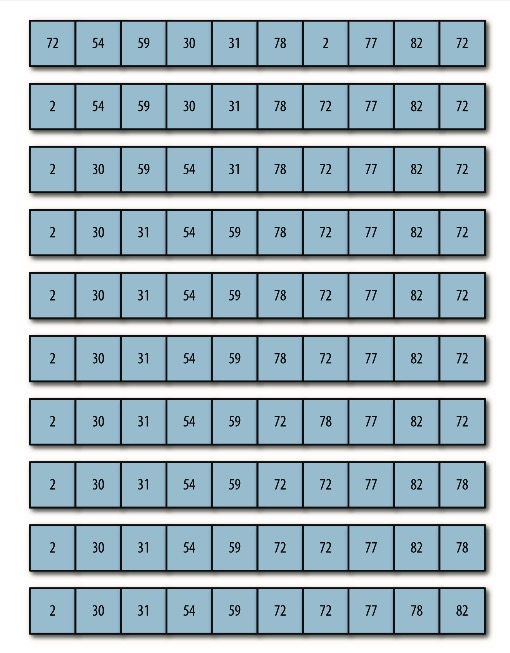``````function selectionSort() {
var min, temp;
for (var outer = 0; outer <= this.dataStore.length-2; ++outer) {
min = outer;
for (var inner = outer + 1;
inner <= this.dataStore.length-1; ++inner) {
if (this.dataStore[inner] < this.dataStore[min]) {
min = inner;
}
swap(this.dataStore, outer, min);
document.write('<br />');
document.write(this.toString());
}
}
}
``````
``````var numElements = 10;
var mynums = new CArray(numElements);
mynums.setData();
mynums.selectionSort();
``````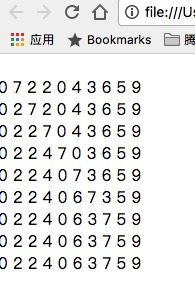#### 插入排序

``````function insertionSort() {
var temp, inner;
for (var outer = 1; outer <= this.dataStore.length - 1; ++outer) {
temp = this.dataStore[outer];
inner = outer;
while (inner > 0 && (this.dataStore[inner - 1] >= temp)) {
this.dataStore[inner] = this.dataStore[inner - 1];
--inner; }
this.dataStore[inner] = temp;
document.write('<br />');
document.write(this.toString());
}
}

``````
``````var numElements = 10;
var mynums = new CArray(numElements);
mynums.setData();
mynums.insertionSort();
``````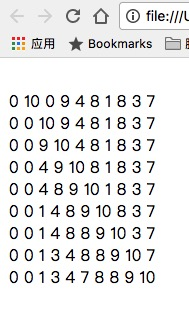#### 基本排序算法的计时比较

``````var start = new Date().getTime();
``````

``````var numElements = 100;    //测试数据100、1000、10000
var nums = new CArray(numElements);
nums.setData();
var start = new Date().getTime();
nums.bubbleSort();
var stop = new Date().getTime();
var elapsed = stop - start;
document.write("对" + numElements + "个元素执行冒泡排序消耗的时间为:" +
elapsed + "毫秒。<br />");
start = new Date().getTime();
nums.selectionSort();
stop = new Date().getTime();
elapsed = stop - start;
document.write("对" + numElements + "个元素执行选择排序消耗的时间为:" +
elapsed + "毫秒。<br />");
start = new Date().getTime();
nums.insertionSort();
stop = new Date().getTime();
elapsed = stop - start;
document.write("对" + numElements + "个元素执行插入排序消耗的时间为:" +
elapsed + "毫秒。");
``````